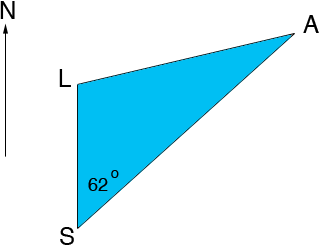SEARCH HOMEMath Central Quandaries & QueriesQuestion from Natalie, a student: Question: A ship spots a lighthouse that is 53m high, at an angle of elevation of 7 degrees that is directly north of the ship. The same ship spots a jet travelling N62E at an altitude of 1500m with an angle of elevation of 15 degrees. How far is the jet from the lighthouse? That's the question. I can't figure out how to draw a diagram to work from. Please help. Thanks, NatalieHi Natalie,

I think that the problem should say "The same ship spots a jet at N62E travelling at an altitude of 1500m with an angle of elevation of 15 degrees." That is the plane is 62 degrees east of north as viewed from the ship. Here is a two dimensional diagram on the surface of the Earth. The ship is at S, the lighthouse at L and the airplane at A.Since you know the height of the lighthouse and the angle of elevation you can use the tangent function to find the length |SL|. Similarly you can find the length |SA|. Now use the law of cosines on the triangle LSA to find |AL|.

I hope this helps,
HarleyMath Central is supported by the University of Regina and The Pacific Institute for the Mathematical Sciences.